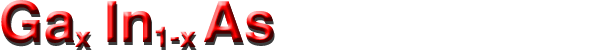## Electrical properties

Basic Parameters
Mobility and Hall Effect
Two-dimensional electron and hole gas mobility in heterostructures
Transport Properties in High Electric Fields
Impact Ionization
Recombination Parameters

### Transport Properties in High Electric Fields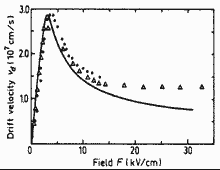Field dependence of the electron drift velocity for Ga0.47In0.53As. T=300 K. Solid line represents Monte Carlo calculation. Points and triangles are experimental data for two samples. Points: no=2.9·1015 cm-3, µo=8500 cm2/Vs. Triangles: no=9µ1014 cm-3, µo=10000 cm2/Vs. Balynas et al. (1990)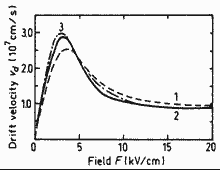Field dependence of the electron drift velocity. T=300 K. Curve 1 - x=0.47 (unstrained) Curve 2 - x=0.22 (strained) Curve 2 - x=0.22 (unstrained). Thobel et al. (1990)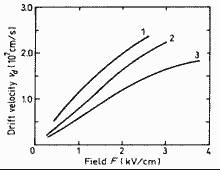Field dependence of the electron drift velocity for Ga0.47In0.53As. T=300 K. Electron concentration no(cm-3): 1 - 1015, 2 - 1017, 3 - 1018. Haase et al. (1985)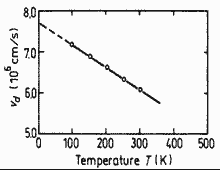Electron saturation velocity versus temperature for Ga0.47In0.53As. Adachi (1992)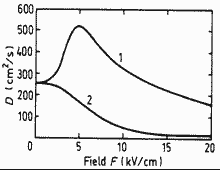The field dependence of longitudinal (1) and transverse (2) diffusion coefficient for Ga0.47In0.53As. Bourel et al.(1991)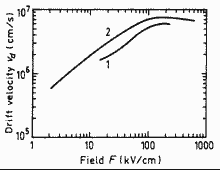Calculated field dependence of the hole drift velocity for x=0.47 (curve 1) and x=1 (curve 2). T=300 K. Adachi (1992)